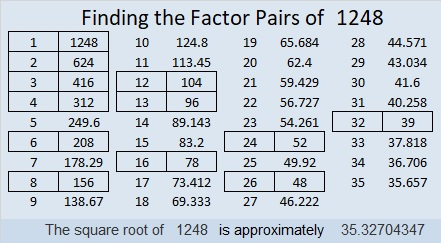# 1248 Factor Trees

It is easy to see that 1248 is divisible by 12 and therefore by 2, 3, 4, and 6, but it actually has a lot more factors than that. I decided to celebrate its many factors by making just a few of its many possible factor trees.Why does 1248 have so many factors? Well, check out its prime factorization:

• 1248 is a composite number.
• Prime factorization: 1248 = 2 × 2 × 2 × 2 × 2 × 3 × 13, which can be written 1248 = 2⁵ × 3 × 13
• The exponents in the prime factorization are 5, 1, and 1. Adding one to each and multiplying we get (5 + 1)(1 + 1)(1 + 1) = 6 × 2 × 2 = 24. Therefore 1248 has exactly 24 factors.
• Factors of 1248: 1, 2, 3, 4, 6, 8, 12, 13, 16, 24, 26, 32, 39, 48, 52, 78, 96, 104, 156, 208, 312, 416, 624, 1248
• Factor pairs: 1248 = 1 × 1248, 2 × 624, 3 × 416, 4 × 312, 6 × 208, 8 × 156, 12 × 104, 13 × 96, 16 × 78, 24 × 52, 26 × 48, or 32 × 39
• Taking the factor pair with the largest square number factor, we get √1248 = (√16)(√78) = 4√78 ≈ 35.327041248 is the sum of four consecutive prime numbers:
307 + 311 + 313 + 317 = 1248

1248 is also the hypotenuse of a Pythagorean triple:
480-1152-1248 which is (5-12-13) times 96

This site uses Akismet to reduce spam. Learn how your comment data is processed.